# Fresnel Equations: What are they? (Derivation & Explanation)

Contents

## What are the Fresnel Equations?

The Fresnel Equations (also known as the Fresnel coefficients) are defined as the ratio of the electric field of a reflected and transmitted wave to the electric field of the incident wave. This ratio is complex and hence, it describes relative amplitude as well as phase shifts between the waves.

The Fresnel Equations (Fresnel coefficients) describe the reflection and transmission of light when it is incident on an interface between two different mediums. The Fresnel Equations were introduced by Augustin-Jean Fresnel. He was the first who understand that the light is a transverse wave.

When the light is incident on the surface of a dielectric, it will be reflected and refracted as a function of angle of incidence. The direction of the reflected wave is given by the “Law of Reflection”.

The Fresnel effect is seen in regular life. It can be seen in shiny as well as rough surfaces also. This effect is very clear on the water surface. When a light incident on water from the air medium, the light will reflect according to the angle of incidence.

The Fresnel effect is everywhere. If you try to look around, you will find many examples. This effect highly depends on the angle of incidence.

The angle of incidence is the angle between the line of sight and surface of the object you are looking for. The below figure shows the effect of the angle of an incident in Fresnel reflection.

## S and P Polarizations

The plane which has the surface normal and the propagation vector of the incoming radiation is known as the plane of incidence or incidence plane.

The plane of incidence plays an important role in the strength of reflection of incident light polarization. The polarization is defined as a property of a transverse wave that specifies the geometrical orientation of the oscillation.

There are two types of polarization;

• S-Polarization
• P-Polarization

When the polarization of light is perpendicular to the plane of the incident, the polarization is known as S-polarization. The ‘S’ word comes from the German word senkrecht that means perpendicular. S-polarization is also known as Transverse Electric (TE).

When the polarization of light is parallel to the plane of an incident or it lying in the plane of an incident. The plane is known as P-Polarization. S-polarization is also known as Transverse Magnetic (TM).

The below figure shows that the incident light is reflected and transmitted in S-polarization and P-Polarization.

## Fresnel Equations Complex Index of Refraction

The Fresnel Equations are a complex equation that means it considers the magnitude and phase both. The Fresnel Equations represents in terms of the electromagnetic field complex amplitude that consider the phase besides power.

These equations are the ratios of an electromagnetic field and it makes in various forms. The complex amplitude coefficients are represented by r and t.

The reflection coefficient ‘r’ is a ratio of electric field complex amplitude of the reflected wave to the incident wave. And the reflection coefficient ‘t’ is a ratio of electric field complex amplitude of the transmitted wave to the incident wave.

As shown in the above figure, we have assumed that the angle of incidence is θi, reflected at an angle of θr, and transmitted at an angle of θt.

Ni is the refractive indices of a medium of incident light and Nt is the refractive indices of a medium of transmitted light.

Hence, there are four Fresnel Equations; two equations for reflection coefficient ‘r’ that is (rp and rs) and two equations for reflection coefficient ‘t’ that is (tp and ts).

## Fresnel Equations Derivation

Let’s assume that incident light reflects as shown in the above figure. In the first case, we will derive a Fresnel Equation for S-Polarization.

For S-Polarization, parallel component E and perpendicular component B is continuous across the boundary between two media.

Hence from the boundary condition, we can write equations for E-field and B-field,

(1)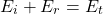(2)We use the below relation between B and E to eliminate B.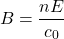And from the law of reflection,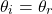Put this value in eq-2,

(3)(4)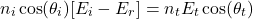(5)(6)(7)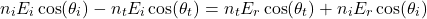(8)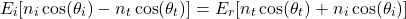(9)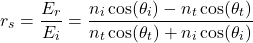Now, for reflection coefficient t, from eq-1 and eq-4,

(10)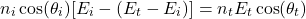(11)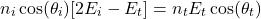(12)(13)(14)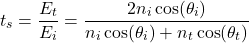These are the Fresnel Equations for perpendicularly polarized light (S-Polarization).

Now, let’s derive equations for parallel polarized light (P-Polarization).

For S-Polarization, equations for E-field and B-field is;

(15)(16)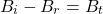We use the below relation between B and E to eliminate B.(17)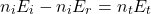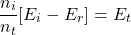Put this value in eq-15,

(18)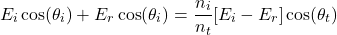(19)(20)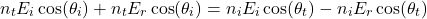(21)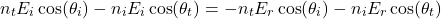(22)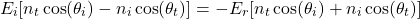(23)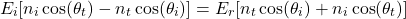(24)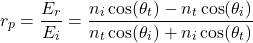Now, for reflection coefficient t, from eq-17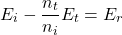Put this value in eq-15

(25)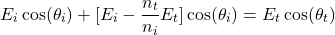(26)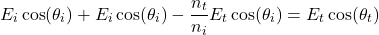(27)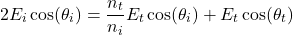(28)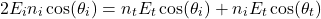(29)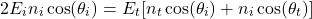(30)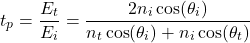Let’s summarized all four Fresnel’s equations,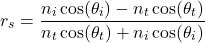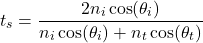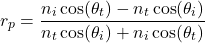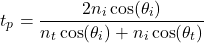Want To Learn Faster? 🎓
Get electrical articles delivered to your inbox every week.
No credit card required—it’s 100% free.